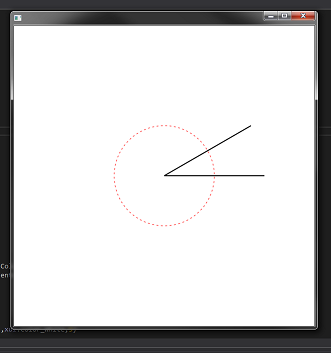# iOS Question How to draw a 30 degree line

Discussion in 'iOS Questions' started by rfresh, Jun 23, 2019.

1. I know how to draw lines. What I need to do is draw a line at a specific angle, such as 30 degrees.

I don't know how to calculate the line angle for the given points of x1, y1, x2, y2?

Thanks...

2. You need to ne more precise on what exactly you want to draw.
x1 and y1 are the coordinates of the beginning point.
Then what limits the end point?
- an x coordinate ? then y2 = y1 + (x2 - x1) * CosD(30)
- a y coordinate ? then x2 = x1 + (y2 - y1) * SinD(30)
- the length of the line ? then
x2 = x1 + Length * CosD(30)
Y2 = y1 + Length * SinD(30)

ilan and Erel like this.
3. here is a b4j code example:

Code:
`#Region Project Attributes    #MainFormWidth: 600    #MainFormHeight: 600#End RegionSub Process_Globals    Type point(x As Float, y As Float)    Private fx As JFX    Private MainForm As Form    Private cnv As B4XCanvas    Private xui As XUIEnd SubSub AppStart (Form1 As Form, Args() As String)    MainForm = Form1    'MainForm.RootPane.LoadLayout("Layout1") 'Load the layout file.    MainForm.Show    Dim center As point    center.Initialize    center.x = MainForm.RootPane.Width/2    center.y = MainForm.RootPane.Height/2      Dim lineLength As Int = 200      cnv.Initialize(MainForm.RootPane)      circleMekuvkav(center,lineLength/2)      cnv.DrawLine(center.x,center.y,center.x+lineLength,center.y,xui.Color_Black,2) '0 deg line    cnv.DrawLine(center.x,center.y,center.x+(lineLength*CosD(-30)),center.y+(lineLength*SinD(-30)),xui.Color_Black,2) '30 degEnd SubSub circleMekuvkav(p As point,r As Int)    cnv.DrawCircle(p.x,p.y,r,xui.Color_Red,False,1)    For i = 0 To 360 Step 5        cnv.DrawLine(p.x,p.y,p.x*((r*2)*CosD(i)),p.y*((r*2)*SinD(i)),xui.Color_White,5)    NextEnd Sub'Return true to allow the default exceptions handler to handle the uncaught exception.Sub Application_Error (Error As Exception, StackTrace As String) As Boolean    Return TrueEnd Sub`#### Attached Files:

• ###### f3.jar
File size:
407 KB
Views:
13
Last edited: Jun 24, 2019
techknight, Erel and José J. Aguilar like this.
4. Thank you Ilan...